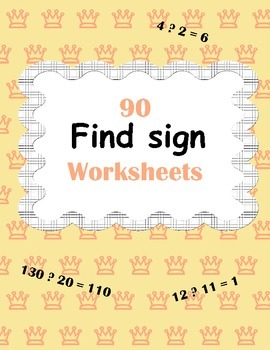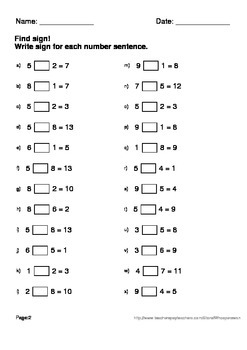## Find sign Worksheets - Plus or Minus\$3.00Buy This Resource Now

Find sign (plus or minus) package includes 90 worksheets.
Answers included.

Table of Contents.
Find sign! Min Number 1. Max Number 9. (30 worksheets)
Example: 7 ? 2 = 5

Find sign! Min Number 1. Max Number 99. (30 worksheets)
Example: 23 ? 30 = 53

Find sign! Min Number 1. Max Number 999. (30 worksheets)
Example: 120 ? 101 = 19

----------------------------------

You May Also Like These Task Cards:
Addition To 10 Task Cards (Free)
2-Digit Addition Task Cards
2-Digit and 1-Digit Addition Task Cards
3-Digit Addition Task Cards
3-Digit and 2-Digit Addition Task Cards
4-Digit Addition Task Cards
Adding Three 2-Digit Addition Task Cards
Adding Three 3-Digit Addition Task Cards
Addition Task Cards Bundle (Bundle)
Subtraction Task Cards (1 digit by 1 digit)
Subtraction Task Cards (2 digit by 2 digit)
Subtraction Task Cards (2 digit by 1 digit)
Subtraction Task Cards (3 digit by 3 digit)
Subtraction Task Cards (3 digit by 2 digit)
Subtraction Task Cards (4 digit by 4 digit)
Subtraction Task Cards Bundle (Bundle)

You May Also Like These Worksheets:
Addition Worksheets
Subtraction Worksheets
Addition and Subtraction
Addition and Subtraction Word Problems
Addition & Subtraction Word Problems Comics
Mystery Pictures (Addition)
Addition Games
Find Sign Worksheets (Plus or minus)

Be the first to know about my new discounts, freebies and product launches:
- Look for the green star next to my store logo and click it to become a follower. You will now receive email updates about this store.
- Follow me on Facebook
- Follow me on Pinterest

File Type:
PDF (Acrobat) Document File
Be sure that you have an application to open this file type before downloading and/or purchasing.
101 pages